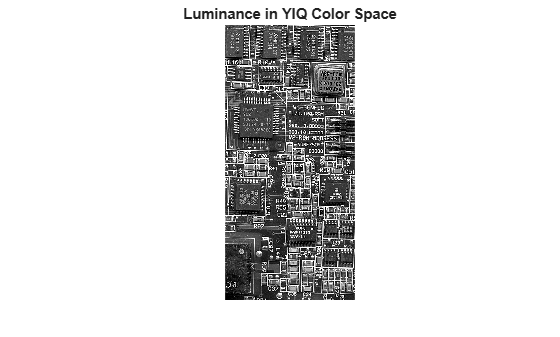# ntsc2rgb

Convert NTSC values to RGB color space

## Syntax

``RGB = ntsc2rgb(YIQ)``
``rgbmap = ntsc2rgb(yiqmap)``

## Description

example

````RGB = ntsc2rgb(YIQ)` converts the luminance (Y) and chrominance (I and Q) values of an NTSC image to red, green, and blue values of an RGB image.```
````rgbmap = ntsc2rgb(yiqmap)` converts an NTSC colormap to an RGB colormap.```

## Examples

collapse all

This example shows how to convert an image from RGB to NTSC color space and back.

Read an RGB image into the workspace.

`RGB = imread('board.tif');`

Convert the image to YIQ color space.

`YIQ = rgb2ntsc(RGB);`

Display the NTSC luminance, represented by the first color channel in the YIQ image.

```imshow(YIQ(:,:,1)) title('Luminance in YIQ Color Space')```Convert the YIQ image back to RGB color space.

`RGB2 = ntsc2rgb(YIQ);`

Display the image that was converted from YIQ to RGB color space.

```figure imshow(RGB2) title('Image Converted from YIQ to RGB Color Space')```## Input Arguments

collapse all

NTSC image, specified as an m-by-n-by-3 numeric array with values in the range [0, 1]. The third dimension of `YIQ` defines the luminance (Y) and chrominance (I and Q) for each pixel, respectively.

Data Types: `double`

NTSC colormap, specified as a `c`-by-3 numeric matrix with values in the range [0, 1]. The value of c corresponds to the number of colors in `rgbmap`. Each row of `yiqmap` is a three-element YIQ triplet that specifies the luminance (Y) and chrominance (I and Q) components of a single color in the colormap.

Data Types: `double`

## Output Arguments

collapse all

RGB image, returned as an m-by-n-by-3 numeric array with values in the range [0, 1]. The third dimension of `RGB` defines the red, green, and blue intensity of each pixel, respectively.

Data Types: `double`

RGB colormap, returned as a c-by-3 numeric matrix with values in the range [0, 1]. Each row of `rgbmap` is a three-element RGB triplet that specifies the red, green, and blue components of a single color in the colormap.

Data Types: `double`

## Algorithms

In the NTSC color space, the luminance is the grayscale signal used to display pictures on monochrome (black and white) televisions. The other components carry the hue and saturation information. The value `0` corresponds to the absence of the component, while the value `1` corresponds to full saturation of the component.

`ntsc2rgb` computes the RGB values from the NTSC components using